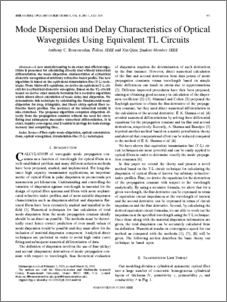# Mode dispersion and delay characteristics of optical waveguides using equivalent TL circuits.

Boucouvalas, A. C. and Qian, X., 2005. Mode dispersion and delay characteristics of optical waveguides using equivalent TL circuits. IEEE Journal of Quantum Electronics, 41 (7), 951-957.

Full text available as:Preview
PDF
Boucouvalas_Output_1.pdf

457kB

## Abstract

A new analysis leading to an exact and efficient algorithm is presented for calculating directly and without numerical differentiation the mode dispersion characteristics of cylindrical dielectric waveguides of arbitrary refractive-index profile. The new algorithm is based on the equivalent transmission-line (T-L) technique. From Maxwell's equations, we derive an equivalent T-L circuit for a cylindrical dielectric waveguide. Based on the TL-circuit model we derive exact analytic formulas for a recursive algorithm which allows direct calculation of mode delay and dispersion. We demonstrate this technique by calculating the fundamental mode dispersion for step, triangular, and linear chirp optical fiber refractive index profiles. The accuracy of the numerical results is also examined. The proposed algorithm computes dispersion directly from the propagation constant without the need for curve fitting and subsequent successive numerical differentiation. It is exact, rapidly convergent, and it results in savings for both storage memory and computing time.

Item Type: Article 0018-9197 Faculty of Science & Technology 949 INVALID USER 12 Dec 2007 14 Mar 2022 13:06